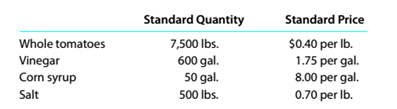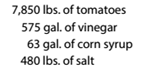Chapter 13, Problem 13.22E

Chapter
Section
Textbook Problem

Standard product cost, direct materials variance HJ. Heinz Company uses standards to control its materials costs. Assume that a batch of ketchup (6,000 pounds) has the following standards:The actual materials in a batch may vary from the standard due to tomato characteristics. Assume that the actual quantities of materials for batch H3001 were as follows:a.Determine the standard unit materials cost per pound for a standard batch. b. Determine the total direct materials quantity variance for batch H3001.

To determine

Concept Introduction:

Direct material variances:

Direct material variances refer to the difference between the standard direct material cost and actual direct material cost incurred. Direct material cost variances are categorized into following two categories:

1. Direct material Rate variance: this variance shows the difference of standard rate and actual rate of material. The formula to calculate this variance is as follows:
2.   Direct material rate variance = (Actual rate – Standard rate) ×Actual Quantity

3. Direct material usage variance: this variance shows the difference of standard usage and actual usage of material. The formula to calculate this variance is as follows:
4.   Direct material usage variance = (Actual Quantity – Standard Quantity)×Standard rate

5. Direct material cost variance: this variance shows the difference of standard cost and actual cost of material. The formula to calculate this variance is as follows:
6.   Direct material cost variance = Direct material rate variance + Direct material usage variance

Requirement-1:

To Calculate:

The Standard unit material cost per pound

Explanation

The Standard unit material cost per pound is calculated as follows:

 Standard Quantity Standard Price Standard Cost per Pound A B C = (A*B)/6000 Whole Tomatoes 7500 $0.40$ 0...
To determine

Concept Introduction:

Direct material variances:

Direct material variances refer to the difference between the standard direct material cost and actual direct material cost incurred. Direct material cost variances are categorized into following two categories:

1. Direct material Rate variance: this variance shows the difference of standard rate and actual rate of material. The formula to calculate this variance is as follows:
2.   Direct material rate variance = (Actual rate – Standard rate) ×Actual Quantity

3. Direct material usage variance: this variance shows the difference of standard usage and actual usage of material. The formula to calculate this variance is as follows:
4.   Direct material usage variance = (Actual Quantity – Standard Quantity)×Standard rate

5. Direct material cost variance: this variance shows the difference of standard cost and actual cost of material. The formula to calculate this variance is as follows:
6.   Direct material cost variance = Direct material rate variance + Direct material usage variance

Requirement-2:

To Calculate:

The Standard unit material cost per pound

Still sussing out bartleby?

Check out a sample textbook solution.

See a sample solution

The Solution to Your Study Problems

Bartleby provides explanations to thousands of textbook problems written by our experts, many with advanced degrees!

Get Started

Find more solutions based on key concepts